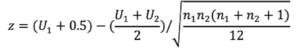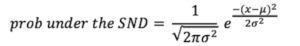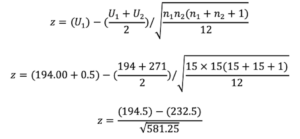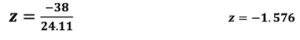Analysis of Non-Parametric Outcomes

# 24 Computing the Wilcoxon-Mann-Whitney U Test

The  Wilcoxon Mann-Whitney is a 2 group non-parametric comparison test equivalent to the Parametric t-test that can be used to test treatment effects when data are not normally distributed.

• The Mann-Whitney U test, which may also be referred to as the Wilcoxon-Mann-Whitney test, or the Wilcoxon Rank-Sum test, evaluates the ranks of the combined scores from two independent groups.
• The Wilcoxon rank-sum test statistic (referred to as Ws if using the name Wilcoxon rank-sum) is based on using the sum of the ranks for observations drawn from one of the groups within the sample of data.

Generally, the groups being studied are designated as GROUP 1 = treatment group and GROUP 2 = control group. This statistic — regardless of whether you refer to it as the Mann-Whitney test or the Wilcoxon rank-sum test, is considered to be among the more powerful of the non-parametric statistical procedures; and when using large samples, the computational result of this test is generally the same as the parametric t-test for two independent groups.

When evaluating the outcome of this statistic, we can test the Wilcoxon rank-sum test statistic against a critical value from a table of standard values, or we can compute a z-score for the comparison of ranks.

In the Mann-Whitney U— Wilcoxon rank-sum test we compute a “z score” (and the corresponding probability of the “z score”) for the sum of the ranks within either the treatment or the control group.  The “U” value in this z formula is the sum of the ranks of the “group of interest” – typically the “treatment group”.

## Essential Formulae

Below is the formula to compute z score for the Wilcoxon-Mann-Whitney test:The formula to compute the probability of arriving at the z that you computed under the standard normal distribution (SND)  is shown in this next formula. We use this probability value to evaluate the outcome of the Mann-Whitney test. In this formula, replace the x term with z from the formula above. The value for π is 3.14, the value for σ2 is 1, the value for μ is 0.## A working example:

You conducted a study to determine if a new treatment procedure was better than the standard method. 30 participants were recruited from a population of students and randomly allocated to either the new treatment procedure (T) or the standard method (S) so that the initial distribution was set at (n1 = 15 and n2 = 15).

After applying the treatments to each group respectively the students were ranked on a specific measure that demonstrates the influence of the two treatment methods. The ranks for each response score are given in the following table while maintaining the student’s group membership.

NT= New treatment; ST = Standard Treatment

 Row 1: Participant ID 1 2 3 4 5 6 7 8 9 10 11 12 13 14 15 Row 2: Dependent Variable Scores 8 12 13 15 19 21 22 28 31 36 37 39 40 41 43 Row 3: Group codes NT NT NT ST ST NT NT ST ST ST NT NT NT NT NT Row 4: Rank of score 1 2 3 4 5 6 7 8 9 10 11 12 13 14 15
 Row 1: Participant ID 16 17 18 19 20 21 22 23 24 25 26 27 28 29 30 Row 2: Dependent Variable Scores 48 52 53 55 59 61 62 68 71 76 77 79 80 81 83 Row 3: Group codes NT NT NT ST ST ST ST ST ST ST ST ST ST NT NT Row 4: Rank of score 16 17 18 19 20 21 22 23 24 25 26 27 28 29 30

Values in Row 2 in the table above represent the score on the dependent variable measuring the response to the two treatment types.

Values in Row 3 in the table above represent the codes for the group membership, where T=new treatment method group and S=standard method group.

Values in Row 3 in the table above represent the Rn= rank of participants within the total data set.

Ranks begin from the lowest score to the highest score.

The sum of the ranks (U1) in the NEW Treatment group are: (1+2+3+6+7+11+12+13+14+15+16+17+18+29+30) = 194

The sum of the ranks (U2) in the STANDARD Treatment group are: (4+5+8+9+10+19+20+21+22+23+24+25+26+27+28) = 271In the case of a tie, we simply organize all of the data as in the table above, and then we assign each observation in a tie its average rank. So if we had two scores 12 and 12 and they had a rank of 3 and 4 then we would simply give the first value of 12 a rank of 3.5 and the second value of 12 a rank of 3.5.

Verifying the Computations with SAS

DATA MWW;

INPUT ID GROUP SCORE @@;

CARDS;

01 1 8 02 1 12 03 1 13 04 2 15 05 2 19 06 1 21 07 1 22 08 2 28 09 2 31

10 2 36 11 1 37 12 1 39 13 1 40 14 1 41 15 1 43 16 1 48 17 1 52 18 1 53

19 2 55 20 2 59 21 2 61 22 2 62 23 2 68 24 2 71 25 2 76 26 2 77 27 2 79

28 2 80 29 1 81 30 1 83

;

PROC PRINT; VAR ID GROUP SCORE;

PROC NPAR1WAY DATA=MWW WILCOXON;

CLASS GROUP; VAR SCORE; EXACT;

RUN;

#### The NPAR1WAY PROCEDURE OUTPUT

Wilcoxon Scores (Rank Sums) for Variable score: Classified by Variable group

 GROUP N Sum of Scores Expected Under H0 Std Dev Under H0 Mean Score New Treatment 15 194.0 232.50 24.109127 12.933333 Standard Treatment 15 271.0 232.50 24.109127 18.066667

Wilcoxon Two-Sample Test: Z includes a continuity correction of 0.5 –> Statistic (S) = 194.00

Normal Approximation: Z = -1.5762; One-Sided Pr < Z = 0.0575; Two-Sided Pr > |Z| = 0.1150.  EXACT TEST: One-Sided Pr <= S = 0.0580; Two-Sided Pr >= |S – Mean| = 0.1160.

## Compute the Sign Test and the Mann-Whitney Test

You are interested in the effects of daily exercise on fitness levels.

You create an experiment in which individuals are allocated to either a twelve-week exercise program or a sedentary control group. You were successful in recruiting 30 subjects, and you matched these individuals on gender and exercise profiles to balance the groups that will either participate or remain sedentary.

You arrange the participants into two groups (n1=15, and n2=15). Group 1 receives a 12-week regimen of noon-hour exercises while Group 2 is considered the control group and does not receive any exercise programming or any related information. Both groups maintain very nearly similar profiles for sleep and diet. The probability of being selected as an exerciser is 50% or “p= ½”, and conversely the probability of being selected to the control group is 50% or “p= ½”

The dependent variable for the experiment is the measure of the individual’s predicted VO2 max as determined by a sub-maximal walking test. Given this scenario and research design, you decide to use the “SIGN TEST” as the statistical procedure to determine if the exercise regimen caused significant changes in the fitness levels of the participants compared to the control group members. The data for this experiment are presented in DATA SET #1 below. COMPLETE THE TABLE, and compute the significance of the sign test.

## Data Set #1 – Computing the significance of the Z statistic in the “Sign test”

 VO2 Grp1 (ml/ kg·min-1) VO2 Grp2 (ml/ kg·min-1) Comparison of VO2 max test scores between the two groups Sign of difference 43 40 Subject1Group1  >  Subject1Group2 + 48 42 Subject2Group1     Subject2Group2 39.4 43.8 Subject3Group1  <  Subject3Group2 – 32.7 31.9 Subject4Group1  >  Subject4Group2 36.9 48.4 Subject5Group1     Subject5Group2 50.2 41.4 Subject6Group1     Subject6Group2 + 39.9 31.9 Subject7Group1     Subject7Group2 45.3 33.2 Subject8Group1     Subject8Group2 40.8 39.6 Subject9Group1    Subject9Group2 39.8 41.2 Subject10Group1  Subject10Group2 45.4 43.5 Subject11Group1  >  Subject11Group2 57.3 52.5 Subject12Group1    Subject12Group2 + 58.7 40.6 Subject13Group1    Subject13Group2 35.4 39.5 Subject14Group1    Subject14Group2 58.4 49.5 Subject15Group1   > Subject15Group2 +
###### The decision concerning the null  hypothesis

Recall that in our study we had N = 30 where we created two matched groups of 15 subjects per group. Treatment group is GROUP 1 and Control group is GROUP 2.

As a follow-up to the study, you decided to compare average resting heart rate responses for the group of individuals who participated in the “lunch-hour exercise group”, against the sedentary control group, over the twelve-week timeline.

The data for this study are presented in Data Set #2 below.  Given the arrangement of data, recall that you are attempting to measure if the heart rates for the “lunch-hour exercise group” are generally higher or lower than the heart rates for the sedentary control group. Since you expect that twelve weeks of exercise at lunch hour should have a positive effect on the cardiovascular system, you also expect that the resting heart rates for the “lunch-hour exercise group” would be generally lower than the heart rates for the sedentary control group.

Use the Mann-Whitney test to compute a “z score” for the sum of the ranks within either the treatment or the control group. Include the null hypothesis and your decision about the null hypothesis based on the computations.

## Data Set #2 – Computing the Mann Whitney Statistic

n1 (the exercise group) = 11 (use EG as the exercising group code).

EG = 89, 95, 103, 105, 109, 113, 114, 115, 117, 123, 128

n2 (the sedentary control group) = 9 (use CG as the control group code)

CG = 100, 101, 107, 119, 126, 134, 135, 136, 139

ROW 1: Scores arranged from lowest to highest

ROW 2: Group membership for scores (E= experimental, C= control)

ROW 3: Rank position for scores (beginning lowest score to highest)

 ROW 1 89 103 113 126 139 ROW 2 E E C E E C C C ROW 3 1 2 4 5 9 15 19 20
 Null hypothesis U1 Sum of ranks in the control group Z Mann-Whitney The decision concerning the null hypothesis